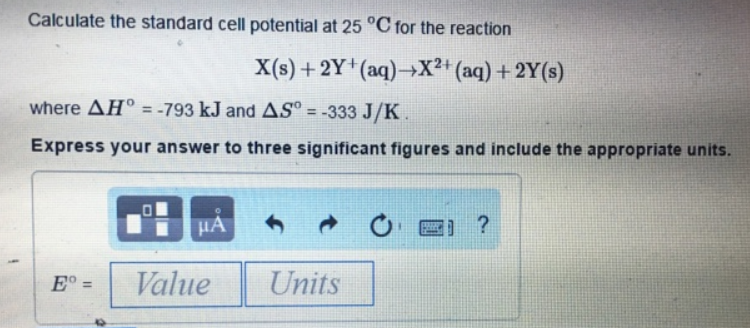# Problem: Calculate the standard cell potential at 25°C for the reactionX(s) + 2Y+(aq) → X2+(aq) + 2Y(s)where ΔH ° = -793 kJ and Δ S° = -333 J/K Express your answer to three significant figures and include the appropriate units

###### FREE Expert Solution
88% (342 ratings)###### Problem Details

Calculate the standard cell potential at 25°C for the reaction

X(s) + 2Y+(aq) → X2+(aq) + 2Y(s)

where ΔH ° = -793 kJ and Δ S° = -333 J/K Express your answer to three significant figures and include the appropriate units